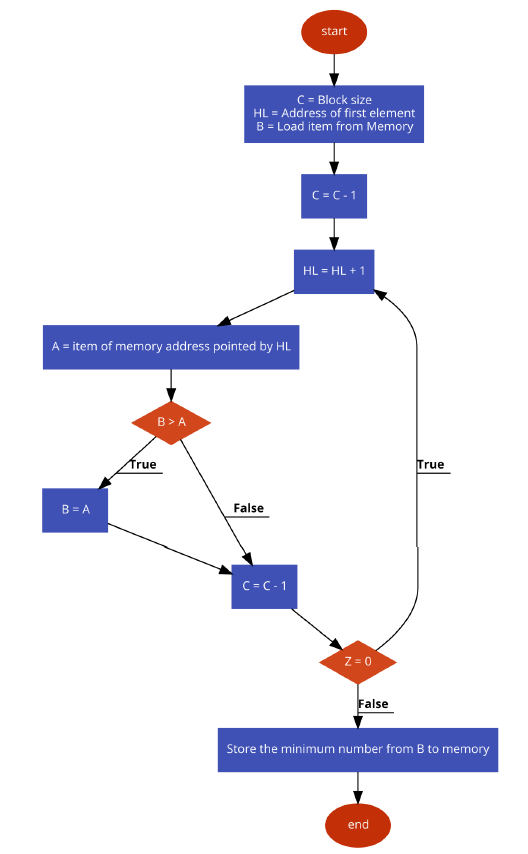# Program to Find the smallest number in an array of data in 8085 Microprocessor

In this program we will see how to find the smallest number from a block of bytes using 8085.

### Problem Statement

Write 8085 Assembly language program to find the smallest number from a block of bytes.

### Discussion

In this program the data are stored at location 8001H onwards. The 8000H is containing the size of the block. After executing this program, it will return the smallest number and store it at location 9000H.

Logic is simple, we are taking the first number at register B to start the job. In each iteration we are getting the number from memory and storing it into register A. Then if B > A, then we simply update the value of Bwith A, otherwise go for the next iteration. Thus we can find the smallest number in a block of bytes.

Data
...
...
8000
06
8001
55
8002
22
8003
44
8004
11
8005
33
8006
66
...
...

### Flow Diagram### Program

HEX Codes
Labels
Mnemonics
F000
21, 00, 80

LXI H,8000H
Point to get array size
F003
4E

MOV C, M
Get the size of array
F004
23

INX H
Point to actual array
F005
46

MOV B, M
Load the first number into B
F006
0D

DCR C
Decrease C
F007
23
LOOP
INX H
Point to next location
F008
7E

MOV A, M
Get the next number from memory to Acc
F009
B8

CMP B
Compare Acc and B
F00A
D2, 0E, F0

JNC SKIP
if B <= A,then skip
F00D
47

MOV B, A
If CY is 1, update B
F00E
0D
SKIP
DCR C
Decrease C
F00F
C2, 07, F0

JNZ LOOP
When count is not 0, go to LOOP
F012
21, 00, 90

LXI H,9000H
F015
70

MOV M, B
Store the minimum number
F016
76

HLT
Terminate the program